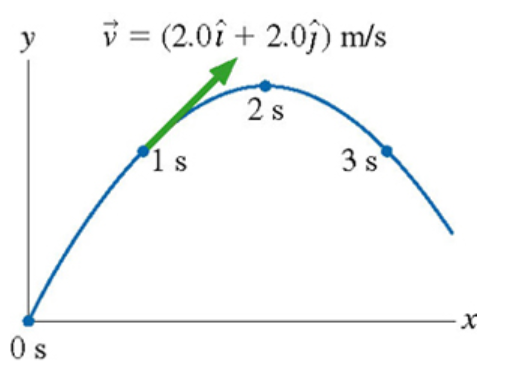# Problem: A physics student on Planet Exidor throws a ball, and it follows the parabolic trajectory shown in the figure. The ball's position is shown at 1s intervals until t=3s. At t=1s , the ball's velocity is v = (2.0 i + 2.0 j) m/s. What is the value of g on planet Exidor?

###### FREE Expert Solution

Uniform accelerated motion (UAM) equations:

We'll use the first UAM equation to determine the gravitational acceleration of the ball.

89% (17 ratings)###### Problem Details

A physics student on Planet Exidor throws a ball, and it follows the parabolic trajectory shown in the figure. The ball's position is shown at 1s intervals until t=3s. At t=1s , the ball's velocity is v = (2.0 i + 2.0 j) m/s.

What is the value of g on planet Exidor?